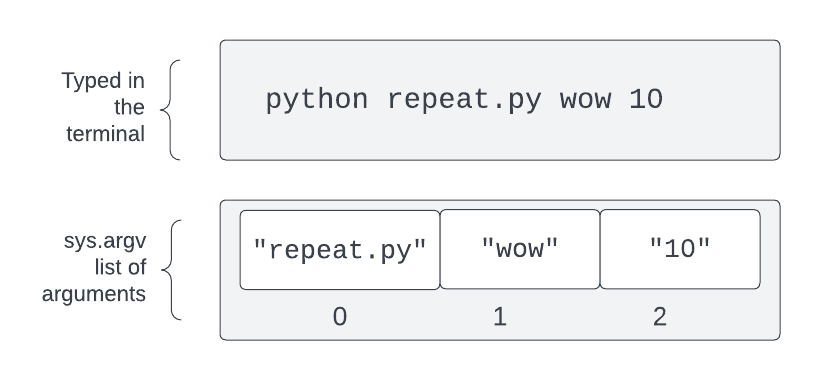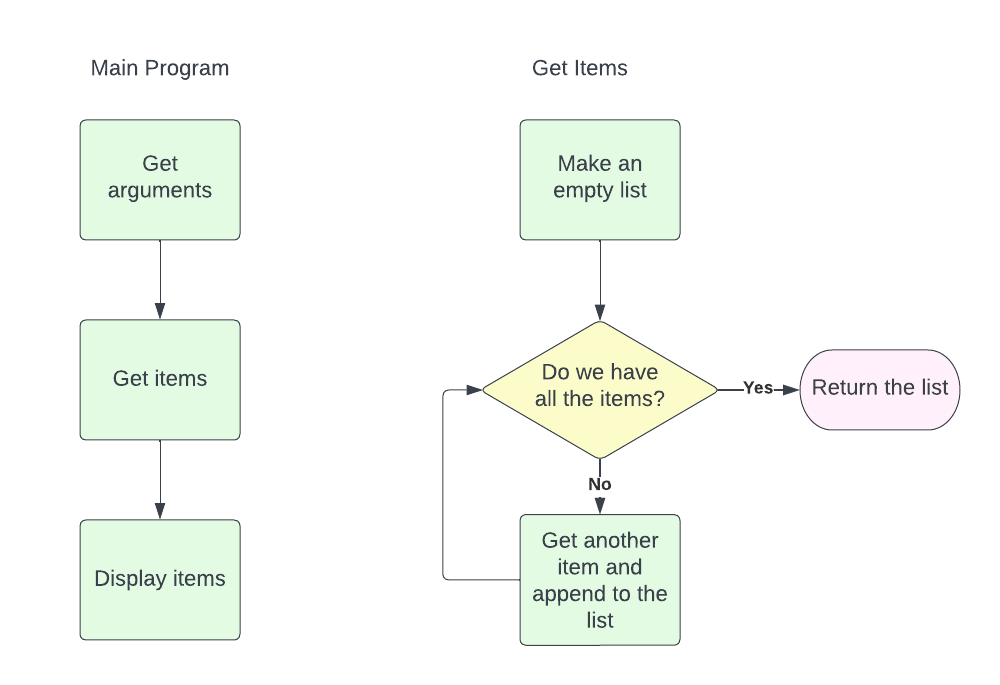Computer Science

# Program arguments

When we run a Python program from the command line, we can give it arguments. Suppose you want a program called “repeat.py” to print “wow” 10 times. You might run it from the terminal like this:

``python repeat.py wow 10``

This will run the program in `repeat.py` and give it these arguments — `wow` and `10`. This program could then print “wow” 10 times.

## argv

To get the arguments into your program, Python puts them into a list called `argv`. To use `argv`, you have to:

``import sys``

Then you can get the arguments in `sys.argv`:

``````import sys

if __name__ == '__main__':
print(sys.argv)``````

In the zip file for this guide you can find a program called `arg_demo.py`. If you download the zip file and put it in your `cs110` directory, then you can open a terminal and run it with:

``````cd arguments
python arg_demo.py first second third``````

then this will print:

``````Your arguments were:
['arg_demo.py', 'first', 'second', 'third']``````

Notice how the first argument in the list is the name of the program. Then the rest of the arguments come after that.

## Accessing individual arguments

Let’s talk about how a Python program gets access to these arguments. Remember, `sys.argv` is a list. We can number all of the items in the list starting from zero:With all lists, we can use indexing to select an item in the list. We do this with square brackets:

``````argument1 = sys.argv
argument2 = sys.argv``````

If you look at `hello.py` you can see this in action:

``````import sys

if __name__ == '__main__':
print(f'Hello {sys.argv}!')``````

This program takes one argument — a name — and says “Hello {name}!“. You can see this by running:

``python hello.py Harry``

You will see:

``````python hello.py Harry
Hello Harry!``````

If you try to run a program that is expecting an argument without any arguments, this causes an error:This is because you tried to access item `1` from the `sys.argv` list, and there is nothing at that index.

You can also run a program with too many arguments, but this won’t cause an error. The extra arguments will just be ignored. Try:

``python hello.py Harry Ron Hermione``

Another example is in `repeat.py`:

``````import sys

def repeat(text, number):
print(text * number)

if __name__ == '__main__':
text = sys.argv
number = int(sys.argv)
repeat(text, number)``````

Every argument in sys.argv is a string. This means we need to convert the second argument into a number if we would like to use it as a number. Then we call a function `repeat()`, which just uses `text * number` to repeat the string that many times. It turns out that you can multiply a string by a number to repeat it! So:## Arguments with spaces in them

What if you want to put spaces in an argument? You need to put quotes around the argument:

``````python repeat.py 'oh wow ' 10
oh wow oh wow oh wow oh wow oh wow oh wow oh wow oh wow oh wow oh wow``````

## Things

To practice these concepts, write a program that prompts a person to input things. After they are done, print the list of things.

The number of things to input and the prompt text should be specified on the command line as arguments. For example:

``````python things.py 3 Fruit
Fruit: apple
Fruit: pear
Fruit: banana

- apple
- pear
- banana``````

### Planning

You will find an empty file in `things.py` where you can write code for this.

Work with a friend to write this code. Try drawing a flow chart.Here is an example flow chart:The main program has three pieces:

• get the arguments (number of things, prompt)
• get the items
• display the items

To get the items, we can accumulate the items into a list:

• make an empty list
• while the list doesn’t have all the items
• get another item
• add it to the list

## Writing code

Let’s write code for the main program:

``````def main(how_many, prompt):
items = get_items(how_many, prompt)
display_items(items)

if __name__ == "__main__":
how_many = int(sys.argv)
prompt = sys.argv
main(how_many, prompt)``````

Some important things to notice:

• we need to convert `how_many` into an integer
• we give both arguments to `main()`
• `main()` calls the next two functions to do steps two and three in the flow chart.

Now we can write `get_items()`:

``````def get_items(how_many, prompt):
items = []
while len(items) < how_many:
item = input(prompt + ': ')
items.append(item)
return items``````

We keep looping as long as the length of the list is less than the desired length, which is given in `how_many`. We use `prompt` to provide the prompt when we call `input()`. Every time we get an item we append it to the list.

We can also write `display_items()`:

``````def display_items(items):
for item in items:
print(f'- {item}')``````

You will need to run this program from the terminal. For example:

``````python things.py 5 'Your Major'